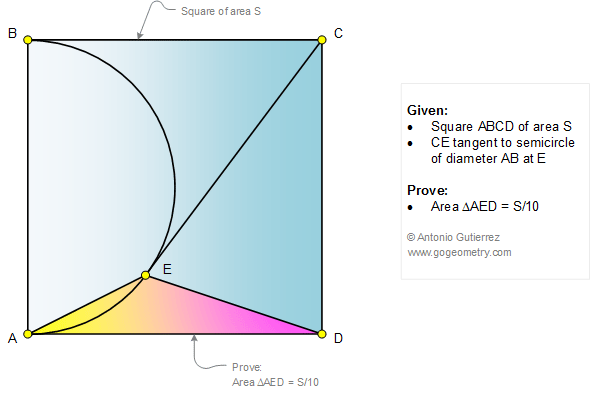Problem 1089. Square, Semicircle, Tangent, Triangle, Area

 < PREVIOUS PROBLEM  |  NEXT PROBLEM > The figure shows a square ABCD of area S. If CE is tangent to semicircle of diameter AB at E, prove that area of triangle AED = S/10.Home | Geometry | Search | Problems | All Problems | Open Problems | Visual Index | 1081-1090 | Square | Circle | Area | Triangle area | Square area | Email | Post a solution / comment | By Antonio Gutierrez